# Lecture 11 Jeans equations and Virial Theorem from Boltzmann Equation II.

## Collisionless Boltzmann Equation summary

The phase space density of mass, or Distribution Function (DF) of the N-body system was defined in Lecture 5 by considering the quantitywheredesignates the mass in the infinitesimal phase-space volumearoundat time. The DFsatisfies the collisionless Boltzmann Equation (CBE),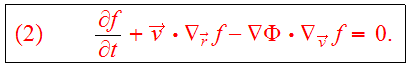The collisionless Boltzmann equation describes the evolution of the distribution functionand it serves as the fundamental equation of collisionless N-body dynamics. In components it is given bywhere the gravitational field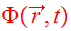is determined self-consistently by Poisson's equation,Eqs. (3,4) may be viewed as a pair of coupled PDEs which together completely describe the evolution of a galaxy.

## Jeans Equations

Moments of the CBE contain important physics about time averages of the dynamical motion. Consider first thevelocity moment of the CBE:The following relation can be used for the mass densityand the relationis valid, if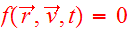for asymptotically large. We introduce the average velocity,and Eq. (5) becomes the continuity equation,Consider now first moments in the velocity components:We use the identityDefinewhich gives the Jeans equation for the first velocity moments:Subtract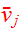times Eq.(9) from Eq.(13) :and definewhich describes the non-streaming motion locally. Eq.(14) becomeswhere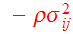is defined as the stress tensor. Eqs.(9,13,15) are known as the Jeans equations.

## Virial Theorems

Multiply Eq.(13) byand integrate over the spatial variables: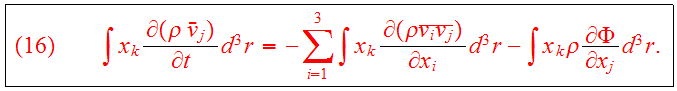We introduce the potential energy tensor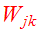: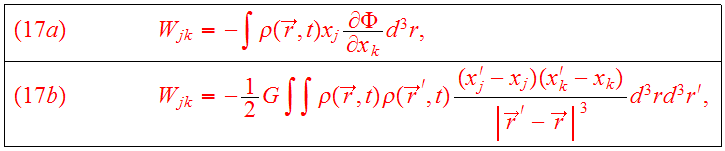symmetric in theindices. The total gravitational potential energy is given by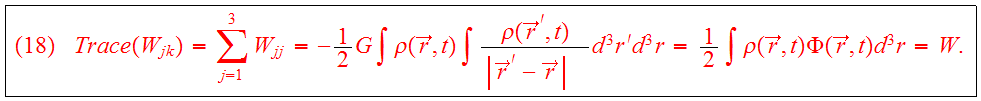The following definition of the kinetic energy tensorwill be used:By averagingand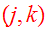in Eq.(16) and using the symmetry properties of the tensors, we getThe moment of inertia tensor is defined asUsing the continuity equation, we findCombining Eqs.(20,22b) we obtain the tensor virial theorem:Sincein steady state,, we get the scalar virial theorem:where M is the total mass of the system,For the total energy, we findandif stars are at rest at infinity;binding energy.

## Jeans Theorem

If the function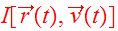is an integral which is conserved along any orbit:we can use the canonical equations to show thatis a steady state soultion of the CBE:Theorem: Any steady-state solution of the CBE depends on the phase-space coordinates only through integrals of motion in the galactic potential, and any function of the integrals yields a steady-state solution of the CBE.

Proof: Suppose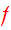is a steady-state solution of CBE. Thenis an integral, so that first part of theorem is true. Conversely, ifto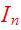areintegrals, thenso thatis an integral and a steady state solution of CBE.